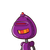# A wire 60m long is bent to a right angled triangle of hypotenuse 26 cm.complete the length of other two sides​

A wire 60m long is bent to a right angled triangle of hypotenuse 26 cm.complete the length of other two sides​

### 1 thought on “A wire 60m long is bent to a right angled triangle of hypotenuse 26 cm.complete the length of other two sides​”

1.hello

ans is 10 and 24

Step-by-step explanation:

Wire length = 60 cm

right triangle is formed

perimeter = 60 cm

hypotenuse = 26

so sum of legs = 60 -26 = 34cm

let one leg be x

other leg = 34-x

(34-x)^2+x^2= 26^2

1156-68x+x^2= 676

2x^2-68x+1156-676=0

2x^2-68x+480=0

/2

x^2-34x+240=0

x^2-24x-10x+240=0

x(x-24)-10(x-24)=0

(x-24)(x-10)=0

The legs are 24 & 10 cm

manasilayanu vijarikkunu

hope it helps u

pls mark as brainlist